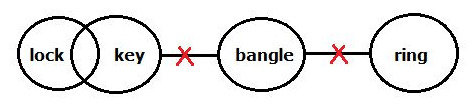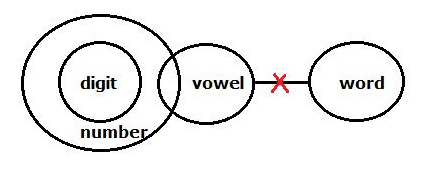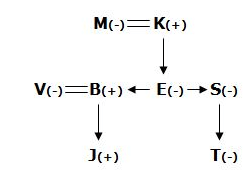# SBI Clerk Pre Reasoning (Day-36)

Dear Aspirants, Our IBPS Guide team is providing new series of Reasoning Questions for SBI Clerk Prelims 2020 so the aspirants can practice it on a daily basis. These questions are framed by our skilled experts after understanding your needs thoroughly. Aspirants can practice these new series questions daily to familiarize with the exact exam pattern and make your preparation effective.

Start Quiz

Ensure Your Ability Before the Exam – Take SBI Clerk 2020 Prelims Free Mock Test

Coding and Decoding:

Direction (1-4): Read the following information carefully and answer the questions given below.

In a certain code language,

‘this is medical shop’ is written as ‘saka di he’,

‘market is near home’ is written as ‘mi kazijo’,

‘home and medical shop’ is written as ‘jo da di he’ and

‘its big market’ is written as ‘faga mi’.

1) How is ‘market’ written in that code language?

a) mi

b) fa

c) zi

d) ga

e) None of these

2) Which of the following is the code for ‘near big shop’?

a) ka mi ga

b) ziga he

c) fazi di

d) Cannot be determined

e) None of these

3) Which of the following is the code for ‘this is home’?

a) kazi di

b) sa mi jo

c) sakajo

d) Cannot be determined

e) None of these

4) What does ‘da’ stands for?

a) market

b) and

c) medical

d) home

e) shop

Syllogism:

Direction (5-6): In each question three statements followed by three conclusions numbered I, II and III have been given. You have to take the given statements to be true even if they seems to be at variance with commonly known facts and then decide which of the given conclusions logically follows from the given statements disregarding commonly known facts.

5) Statements:

No key is a bangle

No ring is a bangle

Some locks are keys

Conclusions:

I) At least some keys are rings

II) At least some locks are bangles

III) Some bangles are rings

None follows

All I, II and III follows

Only III follows

Only I follows

e) None of these

6) Statements:

All digits are numbers

No word is a vowel

Some numbers are vowels

Conclusions:

I) Some digit are word

II) All word can be number

III) At least some word are number

a) Only II and III follows

b) Only I follows

c) All I, II and III follows

d) Only II follows

e) None follows

Blood Relation:

Direction (7-8): Read the following information carefully and answer the questions given below.

Eight persons B, E, J, K, M, S, T and V are in a family with three different generations. J is the son of B. E is the daughter of K and sister of S. M is the mother of E. V is the sister-in-law of S, who has only two siblings. S is the aunt of J. T is the niece of B. E does not has any child.

7) How is K related to T?

a) Granddaughter

b) Uncle

c) Grandfather

d) Father

e) Cannot be determined

8) If J is married to X, then how is X related to E?

a) Daughter

b) Daughter-in-law

c) Niece

d) Sister

e) Cannot be determined

Inequality:

Directions (9-10): In these questions, a relationship between different elements is shown in the statements. The statements are followed by two conclusions. Give answer

a) if only conclusion I is true

b) if only conclusion II is true

c) if either conclusion I or II is true

d) if neither conclusion I nor II is true

e) if both conclusion I and II are true

9) Statements: J ≥ K = L; B < D = N ≥ Q; K ≤ Q.

Conclusions:

I) N ≥ L

II) Q ≥ B

10) Statements: N ≥ P > K ≥ T ; V ≤ W < T = R ; V < X.

Conclusions:

I) R > X

II) W < N

Direction (1-4):Direction (5-6):Direction (7-8):Directions (9-10) :

I) N ≥ L –> N ≥ Q ≥ K = L –> True

II) Q ≥ B –> Q ≤ N = D > B –> False